Solving a Pyraminx or triangle cube is much easier than solving a Rubik’s cube. You just need to understand the notations and the edges to solve it.

While solving any cube, algorithms play a major role. To understand that we have few articles published on Rubik’s cube algorithms. You can learn cube solving easily by following them. You can also follow our YouTube channel where we publish videos on solving different types of cubes by the experts.

In this article, we will be teaching you how to solve the pyraminx cube step by step. All you need to do is follow these steps and learn some algorithms!

So, let's get started!

Notations -

L - Turn the Left part

U - Turn the Top part

R - Turn the Right part

L' - counter clockwise

U'- counter clockwise

R'- counter clockwise

## Step 1 - Solving The Tips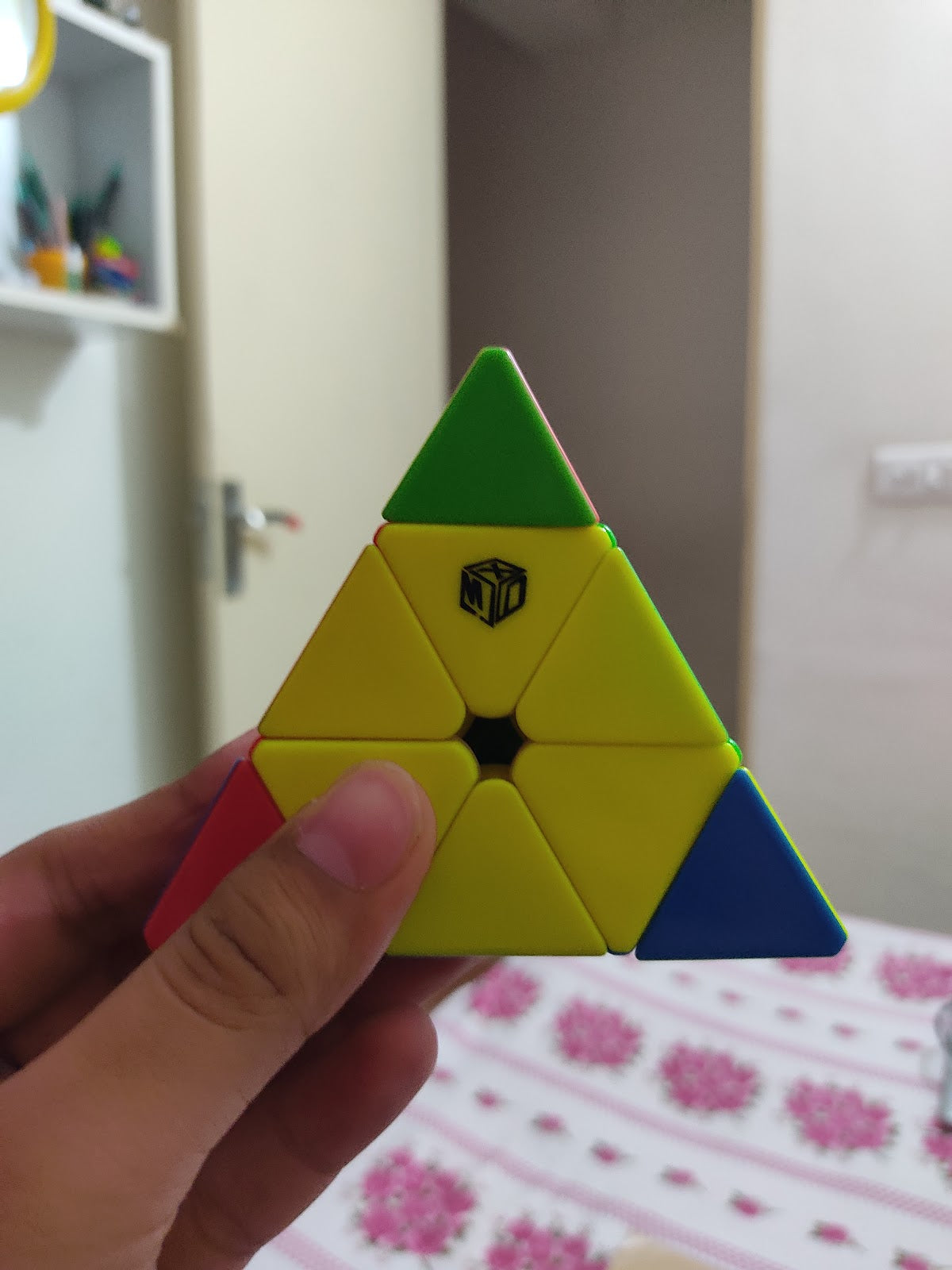In this step we will learn how to solve the tips of the Pyraminx or triangle cube. This is quite intuitive and it’s really easy.

Simply match the  3 tips. This is extremely easy and can be done in 1-2 seconds with practise.

## Step 2 - Solving The Centers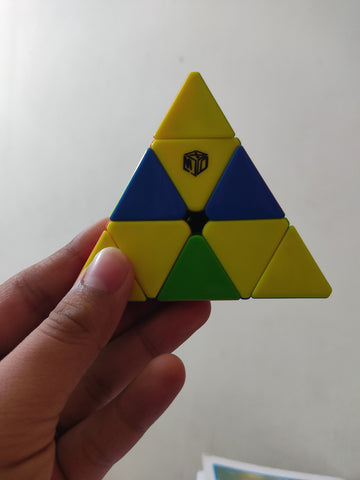Simply turn the 3 layers (U, R and L) until the inner colored pieces get solved.

This is again, a very easy step. Once you get the hang of it, you’ll be able to do this step easily.

## Step 3 – Solve The EdgesNow that we have the centres and tips solved we can move on to solving the rest of the puzzle.

The tips show which colour that side should be.

Move your  Pyraminx until you find the missing edge

Here are 3 cases for this case-

The edge piece to the left.

U' L' U L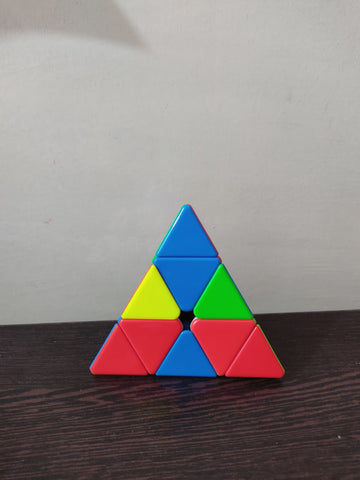The edge piece to the right.

U R U' R'If the edge needs to be flipped.

R U R'

Perform this algorithm and you'll get one of the two cases which we have already shared

This step is a little bit harder than the first 2 steps, but it is still  easy and once you know how to perform the moves correctly then you’ll be able to perform this step with ease.## Step 4 - The Second Layer

The second layer is the last step! For this step you'll have to memorize a set of algorithms for 4 cases.

After solving the first layer you'll get these 4 cases. Perform an algorithm according to the situation and you'll be able to solve the pyraminx easily.

There are 4 cases for this situation.

Clockwise Cycle- L’ U L U L’ UAnti- clockwise cycle- R U’R’U’ R U’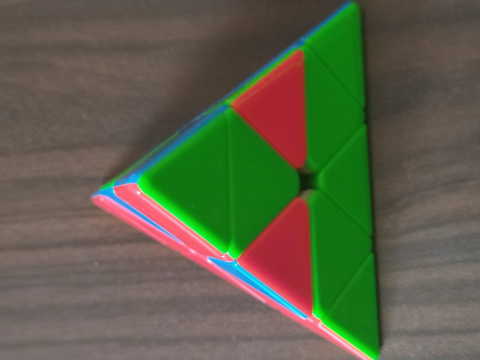Right blocks- L  U R U’ R’ L’Left Blocks- L R U R’ U’ L’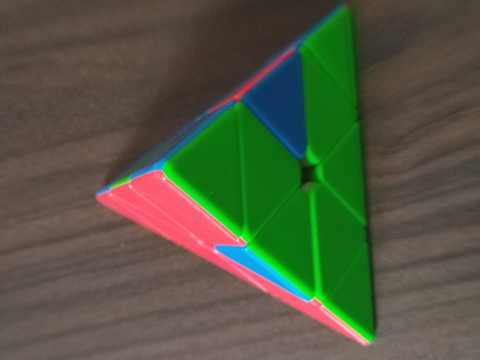Everyone starts out as a beginner and one can only become a master in cubing with practice. My biggest advice would be to practice with focus and improving your finger tricks!

Author - Arush Singh

1 commentGeert Snatersen

very unclear
my last case is not mentioned (i think.
(everything solved except for 2 edges that need to be turned)